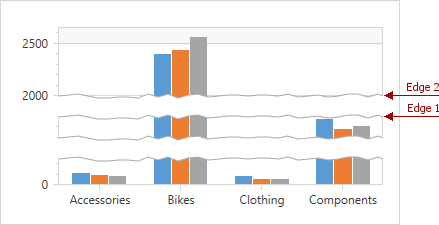# ScaleBreak.Edge1 Property

Gets or sets the scale break’s first edge.

Namespace: DevExpress.Xpf.Charts

Assembly: DevExpress.Xpf.Charts.v21.2.dll

## Declaration

``public object Edge1 { get; set; }``

## Property Value

Type Description
Object

A Object that specifies the scale break’s first edge.

## Remarks

Use the Edge1 and ScaleBreak.Edge2 properties to define a range along an axis, that should be replaced by a scale break. These values expressed in measurement units of an axis.Note that a scale break is successfully created with no regard to whether its edge1 value is more or less than its edge2 value, and to the sequence in which these properties are defined.

## Example

This example demonstrates how to manually create scale breaks for a y-axis.

To do this, add a ScaleBreak object to the Axis2D.ScaleBreaks collection. Then, specify the ScaleBreak.Edge1 and ScaleBreak.Edge2 property values to define a data range that should be replaced by a scale break. Note that the Edge1 and Edge2 values are specified in measurement units of the axis.

``````<dxc:AxisY2D.ScaleBreaks>
<dxc:ScaleBreak Edge1="21000"
Edge2="37000"/>
<dxc:ScaleBreak Edge1="45000"
Edge2="85000" />
</dxc:AxisY2D.ScaleBreaks>
``````